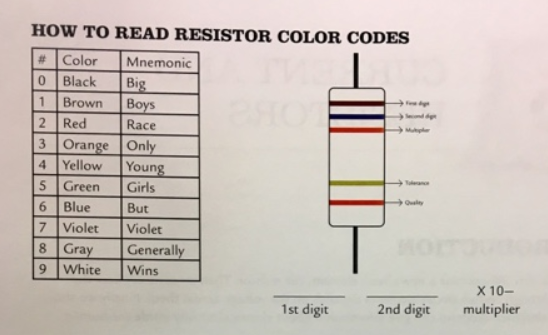# Problem: Resistor color codes:a) What is the resistance of a resistor with the band colors Green Blue Brown? b) What is the resistance of a resistor with the band colors Red Green Black? c) What colors would you see on a 75 kΩ resistor? d) What colors would you see on a 580 Ω resistor?

###### FREE Expert Solution

a)

Green is the first digit = 5

Blue is second digit = 6

Brown is multiplier = × 101 = × 10

82% (462 ratings)###### Problem Details

Resistor color codes:

a) What is the resistance of a resistor with the band colors Green Blue Brown?

b) What is the resistance of a resistor with the band colors Red Green Black?

c) What colors would you see on a 75 kΩ resistor?

d) What colors would you see on a 580 Ω resistor?